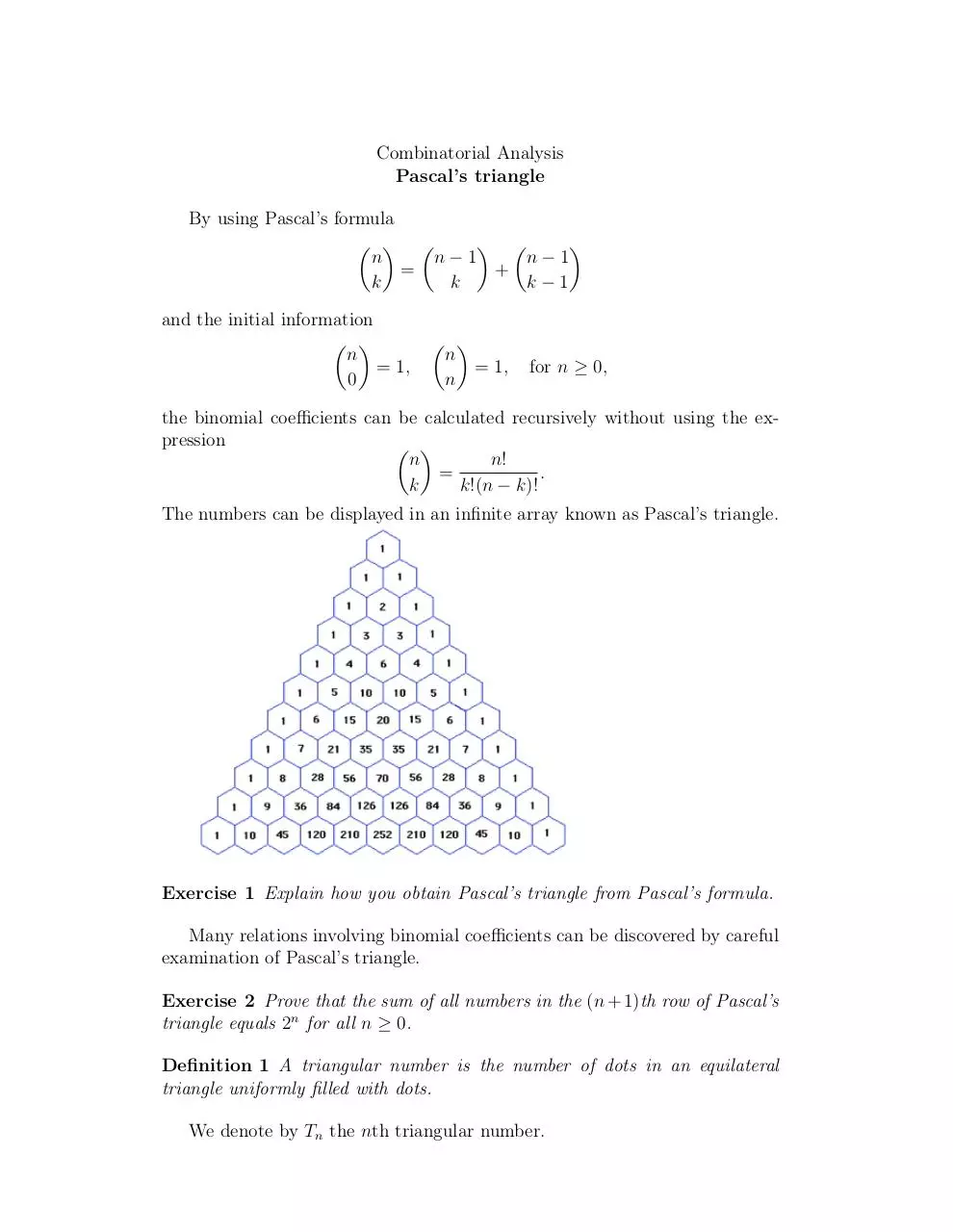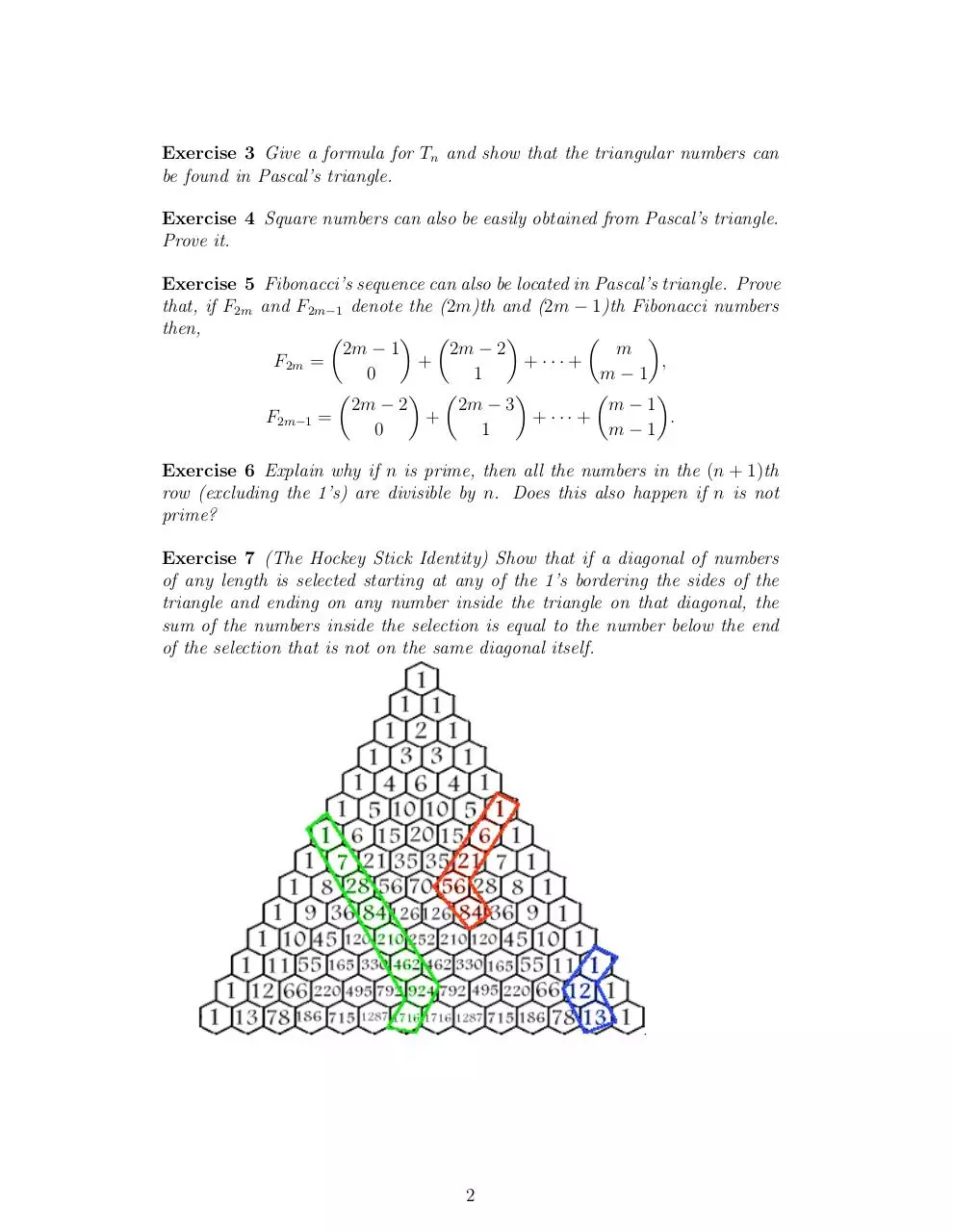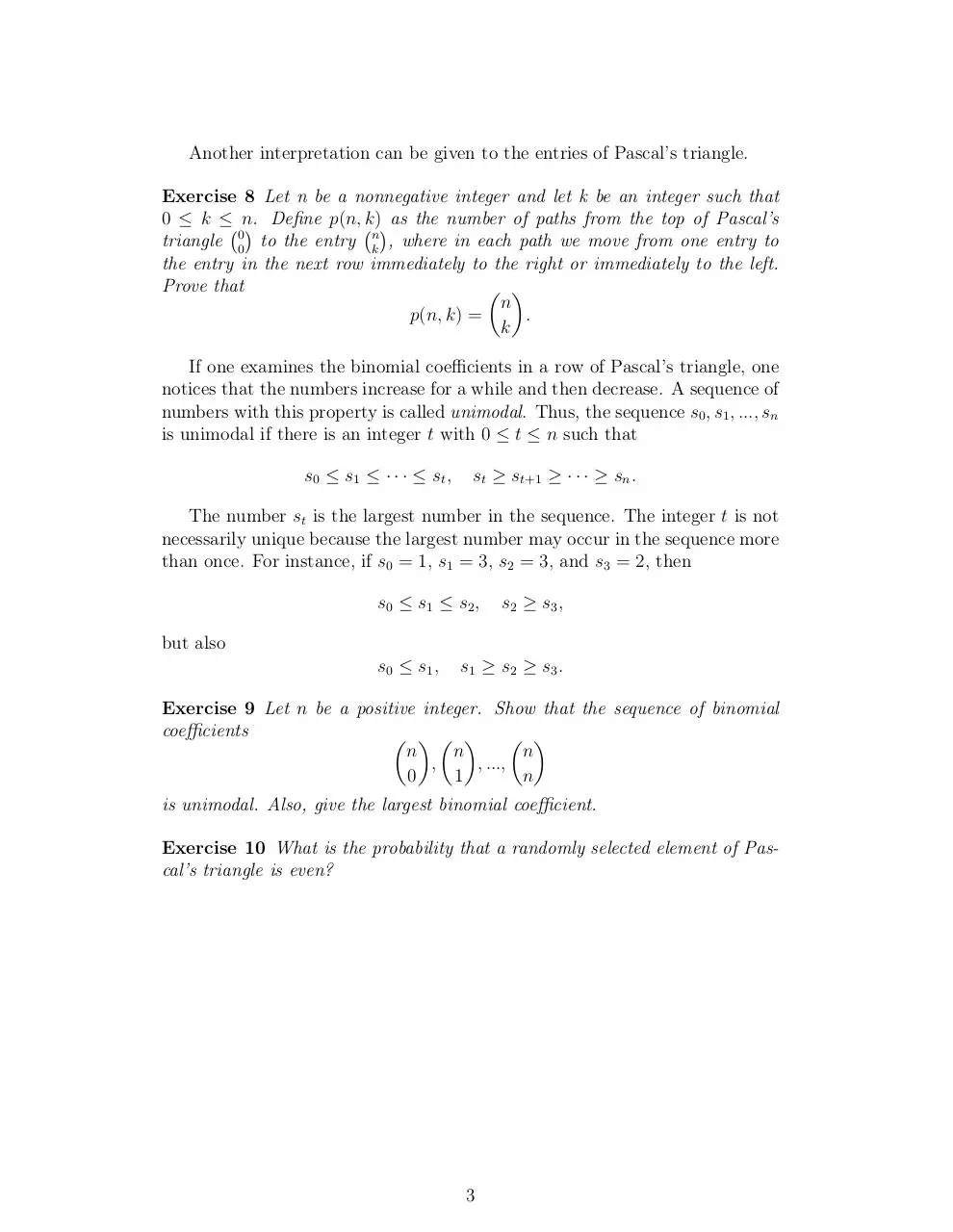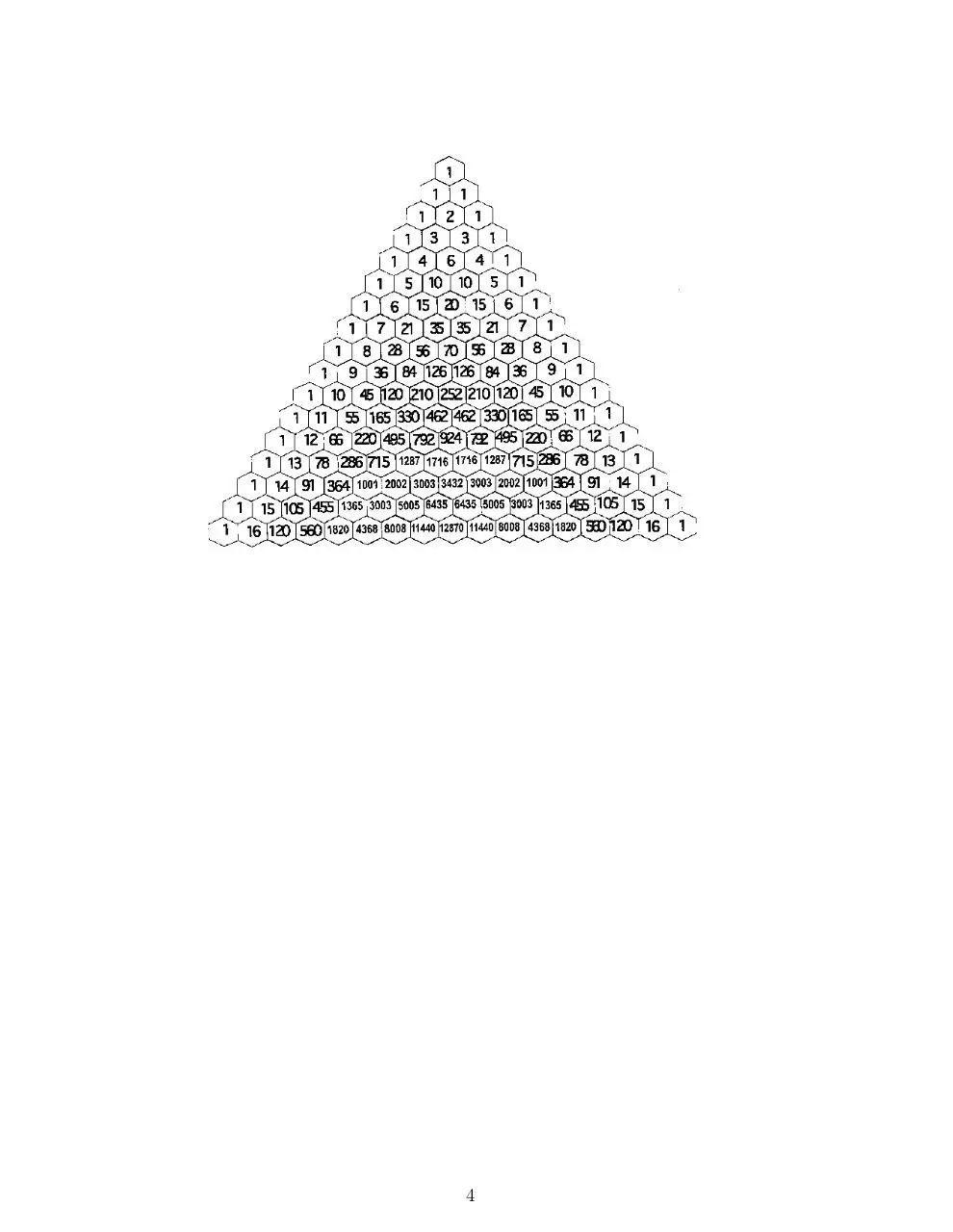# Day 11 Pascals triangle .pdf

### File information

This PDF 1.4 document has been generated by TeX / pdfTeX-1.40.3, and has been sent on pdf-archive.com on 24/01/2014 at 06:10, from IP address 169.231.x.x. The current document download page has been viewed 800 times.
File size: 208.2 KB (4 pages).
Privacy: public file

### Document preview

Combinatorial Analysis
Pascal’s triangle
By using Pascal’s formula
  
 

n
n−1
n−1
=
+
k
k
k−1
and the initial information
 
n
= 1,
0

 
n
= 1,
n

for n ≥ 0,

the binomial coefficients can be calculated recursively without using the expression
 
n
n!
.
=
k
k!(n − k)!
The numbers can be displayed in an infinite array known as Pascal’s triangle.

Exercise 1 Explain how you obtain Pascal’s triangle from Pascal’s formula.
Many relations involving binomial coefficients can be discovered by careful
examination of Pascal’s triangle.
Exercise 2 Prove that the sum of all numbers in the (n + 1)th row of Pascal’s
triangle equals 2n for all n ≥ 0.
Definition 1 A triangular number is the number of dots in an equilateral
triangle uniformly filled with dots.
We denote by Tn the nth triangular number.

Exercise 3 Give a formula for Tn and show that the triangular numbers can
be found in Pascal’s triangle.
Exercise 4 Square numbers can also be easily obtained from Pascal’s triangle.
Prove it.
Exercise 5 Fibonacci’s sequence can also be located in Pascal’s triangle. Prove
that, if F2m and F2m−1 denote the (2m)th and (2m − 1)th Fibonacci numbers
then,

 



2m − 1
2m − 2
m
F2m =
+
+ ··· +
,
0
1
m−1

 



2m − 2
2m − 3
m−1
F2m−1 =
+
+ ··· +
.
0
1
m−1
Exercise 6 Explain why if n is prime, then all the numbers in the (n + 1)th
row (excluding the 1’s) are divisible by n. Does this also happen if n is not
prime?
Exercise 7 (The Hockey Stick Identity) Show that if a diagonal of numbers
of any length is selected starting at any of the 1’s bordering the sides of the
triangle and ending on any number inside the triangle on that diagonal, the
sum of the numbers inside the selection is equal to the number below the end
of the selection that is not on the same diagonal itself.

2

Another interpretation can be given to the entries of Pascal’s triangle.
Exercise 8 Let n be a nonnegative integer and let k be an integer such that
0 ≤ k ≤ n.
 Define p(n, k) as the number of paths from the top of Pascal’s
triangle 00 to the entry nk , where in each path we move from one entry to
the entry in the next row immediately to the right or immediately to the left.
Prove that
 
n
p(n, k) =
.
k
If one examines the binomial coefficients in a row of Pascal’s triangle, one
notices that the numbers increase for a while and then decrease. A sequence of
numbers with this property is called unimodal. Thus, the sequence s0 , s1 , ..., sn
is unimodal if there is an integer t with 0 ≤ t ≤ n such that
s0 ≤ s1 ≤ · · · ≤ st ,

st ≥ st+1 ≥ · · · ≥ sn .

The number st is the largest number in the sequence. The integer t is not
necessarily unique because the largest number may occur in the sequence more
than once. For instance, if s0 = 1, s1 = 3, s2 = 3, and s3 = 2, then
s0 ≤ s1 ≤ s2 ,

s2 ≥ s3 ,

but also
s0 ≤ s1 ,

s1 ≥ s2 ≥ s3 .

Exercise 9 Let n be a positive integer. Show that the sequence of binomial
coefficients
   
 
n
n
n
,
, ...,
0
1
n
is unimodal. Also, give the largest binomial coefficient.
Exercise 10 What is the probability that a randomly selected element of Pascal’s triangle is even?

3

4Day 11-Pascals triangle.pdf (PDF, 208.2 KB)

### Share on social networks

#### HTML Code

Copy the following HTML code to share your document on a Website or Blog